#### Vertex enhancement 

This heuristic strategy focuses effort on vertices that were difficult to connect to other vertices in the roadmap construction algorithm in Figure 5.25. A probability distribution,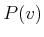, is defined over the vertices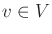. A number of iterations are then performed in which a vertex is sampled from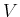according to, and then some random motions are performed from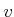to try to reach new configurations. These new configurations are added as vertices, and attempts are made to connect them to other vertices, as selected by the NEIGHBORHOOD function in an ordinary iteration of the algorithm in Figure 5.25. A recommended heuristic  for definingis to define a statistic for eachas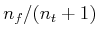, in which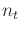is the total number of connections attempted for, and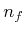is the number of times these attempts failed. The probabilityis assigned as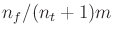, in which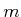is the sum of the statistics over all(this normalizes the statistics to obtain a valid probability distribution).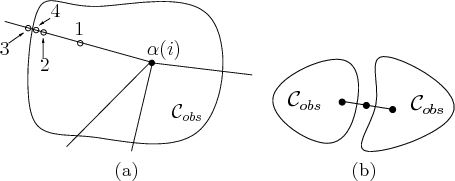Steven M LaValle 2020-08-14